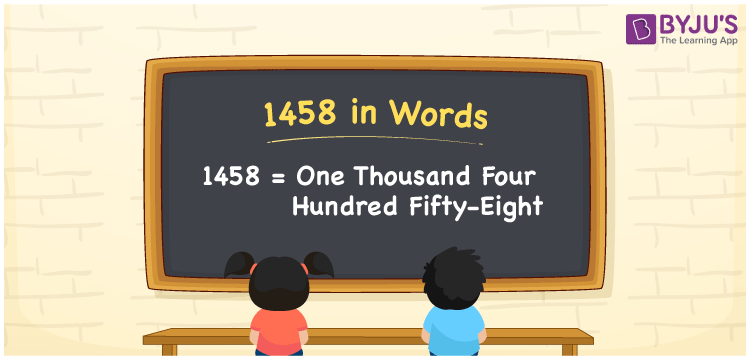# 1458 in Words

One thousand four hundred fifty-eight is the number name of 1458. It is very easy to convert the numbers into words if you know the place value system. We know that 1458 is a natural number that lies between the numbers 1457 and 1459. Also, 1458 is a cardinal number, as it specifies the number of objects equivalent to the value of one thousand four hundred fifty-eight.

 1458 in Words: One Thousand Four Hundred Fifty-eight. One Thousand Four Hundred Fifty-eight in Numerical Form: 1458.

## 1458 in English Words## How to Write 1458 in Words?

Below is the place value table for the number 1458:

 Thousands Hundreds Tens Ones 1 4 5 8

The expanded form of 1458 is as follows:

= 1 × Thousand + 4 × Hundred + 5 × Ten + 8 × One

= 1 × 1000 + 4 × 100 + 5 × 10 + 8 × 1

= 1000 + 400 + 50 + 8

= 1458

= One thousand four hundred fifty-eight

Hence, 1458 in words is one thousand four hundred fifty-eight.

1458 in words – One thousand four hundred fifty-eight

Is 1458 an odd number? – No

Is 1458 an even number? – Yes

Is 1458 a perfect square number? – No

Is 1458 a perfect cube number? – No

Is 1458 a prime number? – No

Is 1458 a composite number? – Yes

## Frequently Asked Questions on 1458 in Words

Q1

### How to spell 1458 in words?

1458 in words is one thousand four hundred fifty-eight.

Q2

### Simplify 1400 + 58, and express it in words.

Simplifying 1400 + 58, we get 1458. Hence, 1458 in words is one thousand four hundred fifty-eight.

Q3

### Is 1458 a prime number?

No, 1458 is not a prime number.